Author: Oscar Cronquist Article last updated on October 21, 2022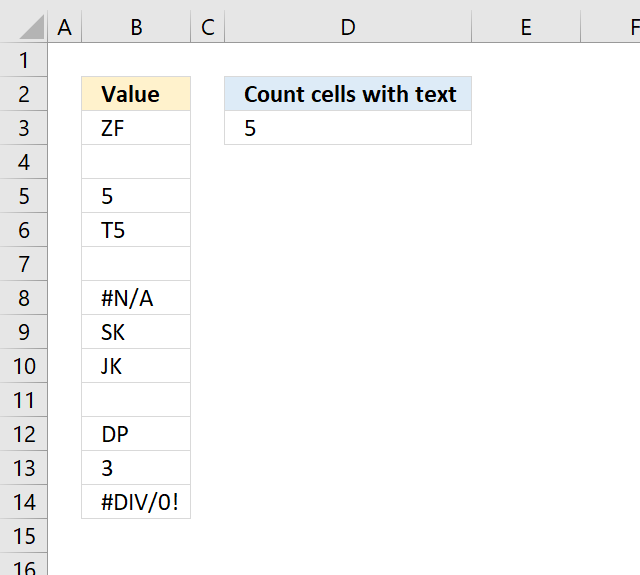## 1. Count cells with textThe following formula in cell D3 counts cells with values stored as text.

=SUMPRODUCT(ISTEXT(B3:B14)*1)

In other words, cells containing nothing, errors, boolean values, and numbers are not counted.

Numbers stored as text are counted, as well as cells containing a space a character or more.

### 1.1 Explaining formula

#### Step 1  - Identify values stored as text

The ISTEXT function returns TRUE or FALSE depending on if a cell has a value stored as text.

ISTEXT(B3:B14)

becomes

ISTEXT({"ZF"; 0; 5; "T5"; 0; #N/A; "SK"; "JK"; 0; "DP"; 3; #DIV/0!})

and returns

{TRUE; FALSE; FALSE  ... }.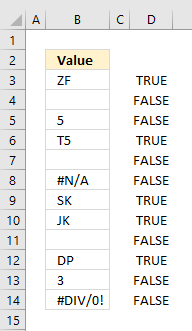#### Step 2 - Convert boolean values to numbers

To count boolean values (TRUE, FALSE) we need to convert them into numbers. TRUE = 1 and FALSE = 0 (zero).

ISTEXT(B3:B14)*1

becomes

{TRUE; FALSE; FALSE  ... }*1

and returns

{1; 0; 0; ... }.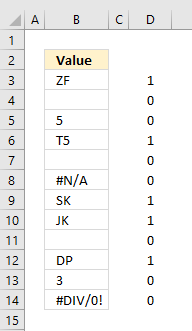#### Step 3 - Count numbers

The SUMPRODUCT function has a great advantage over the SUM function, in most cases, you don't need to enter the formula as an array formula if you are working with arrays.

SUMPRODUCT(ISTEXT(B3:B14)*1)

becomes

SUMPRODUCT({1;0;0;1;0;0;1;1;0;1;0;0})

and returns 5 in cell D3.

1+0+0+1+0+0+1+1+0+1+0+0 = 5.

## 2. Count cells with text excluding cells containing a space character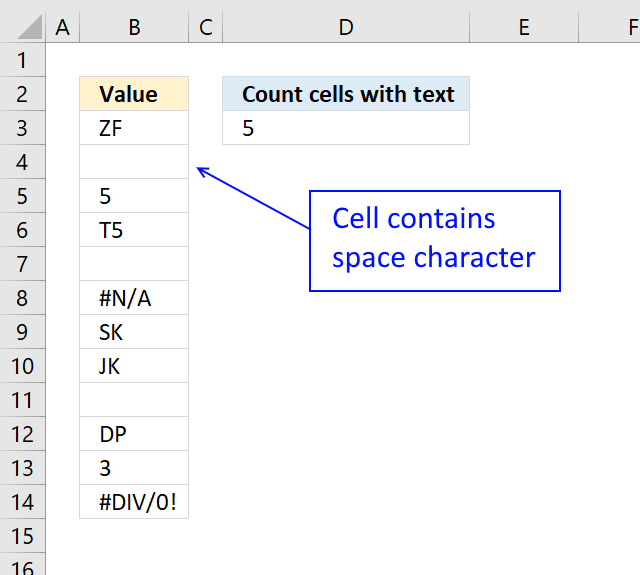Cell B4 contains a space character, the array formula below does not count cells containing a space character.

=SUM(IF(ISTEXT(B3:B14),(B3:B14<>" "),0)*1)

B3:B14<>" "  makes sure that cells containing a space character are not counted.

### 2.1 How to enter an array formula

To enter an array formula, type the formula in a cell then press and hold CTRL + SHIFT simultaneously, now press Enter once. Release all keys.

The formula bar now shows the formula with a beginning and ending curly bracket telling you that you entered the formula successfully. Don't enter the curly brackets yourself.

### 2.2 Explaining formula

#### Step 1 - Check if a value is text

The ISTEXT function returns TRUE if argument is text.

Function syntax: ISTEXT(value)

ISTEXT(B3:B14)

becomes

ISTEXT({"ZF"; " "; 5; "T5"; 0; #N/A; "SK"; "JK"; 0; "DP"; 3; #DIV/0!})

and returns

{TRUE; TRUE; FALSE; TRUE; FALSE; FALSE; TRUE; TRUE; FALSE; TRUE; FALSE; FALSE}.

#### Step 2 - Check if a value is equal to a space character

The less than and greater than characters let you evaluate "not equal to" between two or more values.

B3:B14<>" "

becomes

{"ZF"; " "; 5; "T5"; 0; #N/A; "SK"; "JK"; 0; "DP"; 3; #DIV/0!}<>" "

and returns

{TRUE; FALSE; TRUE; TRUE; TRUE; #N/A; TRUE; TRUE; TRUE; TRUE; TRUE; #DIV/0!}

#### Step 3 - Replace text values with the result of a logical test

The IF function returns one value if the logical test is TRUE and another value if the logical test is FALSE.

Function syntax: IF(logical_test, [value_if_true], [value_if_false])

IF(ISTEXT(B3:B14),(B3:B14<>" "),0)

becomes

IF({TRUE; TRUE; FALSE; TRUE; FALSE; FALSE; TRUE; TRUE; FALSE; TRUE; FALSE; FALSE},{TRUE; FALSE; TRUE; TRUE; TRUE; #N/A; TRUE; TRUE; TRUE; TRUE; TRUE; #DIV/0!},0)

and returns

{TRUE; FALSE; 0; TRUE; 0; 0; TRUE; TRUE; 0; TRUE; 0; 0}.

#### Step 4 - Multiply by 1 to convert boolean values to their numerical equivalents

The asterisk character lets you multiply numbers in an Excel formula.

IF(ISTEXT(B3:B14),(B3:B14<>" "),0)*1

becomes

{TRUE; FALSE; 0; TRUE; 0; 0; TRUE; TRUE; 0; TRUE; 0; 0}*1

and returns

{1; 0; 0; 1; 0; 0; 1; 1; 0; 1; 0; 0}.

#### Step 5 - Add numbers and return a total

The SUM function allows you to add numerical values, the function returns the sum in the cell it is entered in. The SUM function is cleverly designed to ignore text and boolean values, adding only numbers.

Function syntax: SUM(number1, [number2], ...)

SUM(IF(ISTEXT(B3:B14),(B3:B14<>" "),0)*1)

becomes

SUM({1; 0; 0; 1; 0; 0; 1; 1; 0; 1; 0; 0})

and returns 5.

## 3. Count text values returned from an Excel function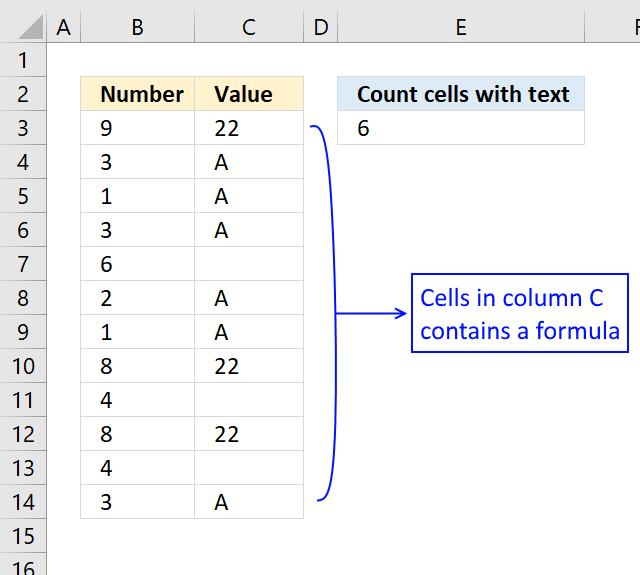The formula below lets you count text values returned from an Excel function.

=SUMPRODUCT(ISTEXT(C3:C14)*(C3:C14<>""))

The ISTEXT function handles empty values from an Excel function as a text value. To avoid counting those non-values I simply use the smaller than and larger than signs <> meaning not equal to.

### 3.1 Explaining formula

#### Step 1 - Check if value is text

The ISTEXT function returns TRUE if argument is text.

Function syntax: ISTEXT(value)

ISTEXT(C3:C14)

becomes

ISTEXT({22; "A"; "A"; "A"; ""; "A"; "A"; 22; ""; 22; ""; "A"})

and returns

{FALSE; TRUE; TRUE; TRUE; TRUE; TRUE; TRUE; FALSE; TRUE; FALSE; TRUE; TRUE}.

#### Step 2 - Check if a value is not empty

The less than and greater than characters lets you evaluate if a value is "not equal to" another value, the result is a boolean value TRUE or FALSE.

C3:C14<>""

becomes

{22; "A"; "A"; "A"; ""; "A"; "A"; 22; ""; 22; ""; "A"}<>""

and returns

{TRUE; TRUE; TRUE; TRUE; FALSE; TRUE; TRUE; TRUE; FALSE; TRUE; FALSE; TRUE}.

#### Step 3 - Multiply arrays (AND logic)

ISTEXT(C3:C14)*(C3:C14<>"")

becomes

{FALSE; TRUE; TRUE; TRUE; TRUE; TRUE; TRUE; FALSE; TRUE; FALSE; TRUE; TRUE}*{TRUE; TRUE; TRUE; TRUE; FALSE; TRUE; TRUE; TRUE; FALSE; TRUE; FALSE; TRUE}

and returns

{0; 1; 1; 1; 0; 1; 1; 0; 0; 0; 0; 1}.

#### Step 4 - Add values and return a total

The SUMPRODUCT function calculates the product of corresponding values and then returns the sum of each multiplication.

Function syntax: SUMPRODUCT(array1, [array2], ...)

SUMPRODUCT(ISTEXT(C3:C14)*(C3:C14<>""))

becomes

SUMPRODUCT({0; 1; 1; 1; 0; 1; 1; 0; 0; 0; 0; 1})

and returns 6.

## 4. Count text values excluding numbers stored as text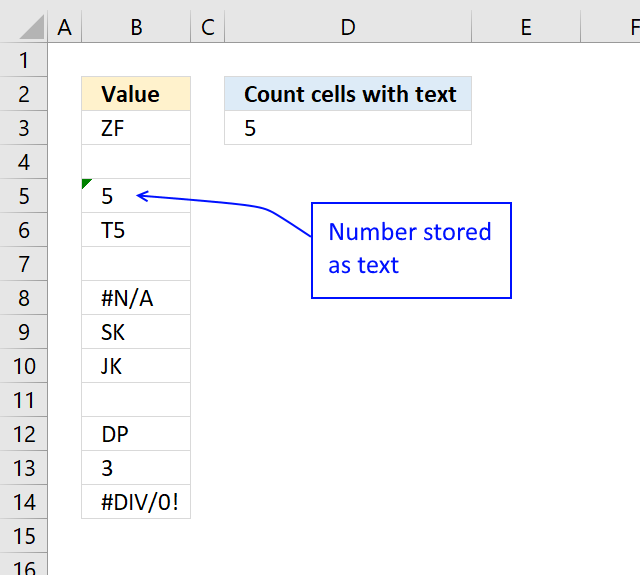This formula counts cells containing text values excluding numbers stored as text from the count.

Cell B5 contains a number stored as text, to exclude that number from the count use the following formula:

=SUMPRODUCT(ISTEXT(B3:B14)*NOT(ISNUMBER(B3:B14*1)))

### 4.1 Explaining formula

#### Step 1 - Check if a value is text

The ISTEXT function returns TRUE if argument is text.

Function syntax: ISTEXT(value)

ISTEXT(B3:B14)

becomes

ISTEXT({"ZF";0;"5";"T5";0;#N/A;"SK";"JK";0;"DP";3;#DIV/0!})

and returns

{TRUE; FALSE; TRUE; TRUE; FALSE; FALSE; TRUE; TRUE; FALSE; TRUE; FALSE; FALSE}.

#### Step 2 - Check if a value is a number

The asterisk character lets you multiply numbers in an Excel formula. It returns a product if both values are numbers and an error if not.

It also converts numbers stored as text like "5" to 5.

B3:B14*1

becomes

{"ZF";0;"5";"T5";0;#N/A;"SK";"JK";0;"DP";3;#DIV/0!}*1

and returns

{#VALUE!; 0; 5; #VALUE!; 0; #N/A; #VALUE!; #VALUE!; 0; #VALUE!; 3; #DIV/0!}.

#### Step 3 - Check if a value is a number

The ISNUMBER function checks if a value is a number, returns TRUE or FALSE.

Function syntax: ISNUMBER(value)

ISNUMBER(B3:B14*1)

becomes

ISNUMBER({#VALUE!; 0; 5; #VALUE!; 0; #N/A; #VALUE!; #VALUE!; 0; #VALUE!; 3; #DIV/0!})

and returns

{FALSE; TRUE; TRUE; FALSE; TRUE; FALSE; FALSE; FALSE; TRUE; FALSE; TRUE; FALSE}.

#### Step 4 - Perform boolean opposite

The NOT function returns the boolean opposite to the given argument.

Function syntax: NOT(logical)

NOT(ISNUMBER(B3:B14*1))

becomes

NOT({FALSE; TRUE; TRUE; FALSE; TRUE; FALSE; FALSE; FALSE; TRUE; FALSE; TRUE; FALSE})

and returns

{TRUE; FALSE; FALSE; TRUE; FALSE; TRUE; TRUE; TRUE; FALSE; TRUE; FALSE; TRUE}.

#### Step 5 - Multiply arrays

The asterisk character lets you also multiply boolean values, this performs AND logic on two arrays. AND logic returns TRUE if both values are TRUE.

TRUE * TRUE = TRUE (1)
TRUE * FALSE = FALSE (0)
FALSE * FALSE = FALSE (0)

The multiplication also converts boolean values to their numerical equivalents.

TRUE - 1
FALSE - 0 (zero)

ISTEXT(B3:B14)*NOT(ISNUMBER(B3:B14*1))

becomes

{TRUE; FALSE; TRUE; TRUE; FALSE; FALSE; TRUE; TRUE; FALSE; TRUE; FALSE; FALSE} * {TRUE; FALSE; FALSE; TRUE; FALSE; TRUE; TRUE; TRUE; FALSE; TRUE; FALSE; TRUE}

and returns

{1; 0; 0; 1; 0; 0; 1; 1; 0; 1; 0; 0}

#### Step 6 - Add values and return a total

The SUMPRODUCT function calculates the product of corresponding values and then returns the sum of each multiplication.

Function syntax: SUMPRODUCT(array1, [array2], ...)

SUMPRODUCT(ISTEXT(B3:B14)*NOT(ISNUMBER(B3:B14*1)))

becomes

SUMPRODUCT({1; 0; 0; 1; 0; 0; 1; 1; 0; 1; 0; 0})

and returns

5. 1 + 1 + 1 + 1 + 1 = 5